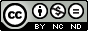Resolución acoplada de las ecuaciones de Navier-Stokes mediante una implementación parcialmente implícita de la Interpolación del Momento.

Cubero García, Ana
Fueyo Díaz, Norberto (dir.)

ISBN: 978-84-692-4370-1

Abstract: In this work, a new algorithm for solving the Navier-Stokes equations in a coupled and implicit manner is proposed; it combines techniques alternatively used in the two traditional approaches in the field (i.e. methods based on pressure, implicit and segregated, and methods based on density, explicit and coupled). In order to improve the conditioning of the matrix of the coupled system, an equation for pressure is derived from the equations of continuity and momentum, and a term of artificial compressibility is added to the resulting Poisson equation. The Navier-Stokes equations are discretized according to the finite volume method and the co-located grid scheme. To avoid the well-known phenomenon of velocity-pressure decoupling that occurs in this kind of grids, the velocity components of the mass flow through the corresponding faces are calculated following the Momentum Interpolation method. This work makes use of a new formulation for this interpolation which avoids some of the inconsistencies in the original expression; it also allows to obtain a compact Poisson equation (for pressure), as well as its implementation in a partially implicit way, thus achieving the velocity-pressure coupling in the same (current) iteration, without increasing the size of the computational molecule. The solution of a wide range of benchmark cases, by means of a parallel CFD code also developed along this research, confirms the good behaviour of the algorithm regarding accuracy, robustness, and numerical stability. It is found adequate for both incompressible, highly convection-dominated flows and those where strong temperature gradients and variable density are present. Finally, the capacity of the proposed algorithm for solving a computationally demanding problem is shown: the simulation of the three dimensional, transient and turbulent vortex shedding at a moderately high Reynolds number is performed using Large Eddy Simulation (LES).

Pal. clave: Pressure-based coupled solver ; Momentum Interpolation ; Preconditioning ; Large Eddy Simulation ; Parallel CFD code.

Knowledge area: Mecánica de fluidos

Department: Ciencia y Tecnología de Materiales y Fluidos: Mecánica de Fluidos

Nota: Tesis-Univ. Zaragoza